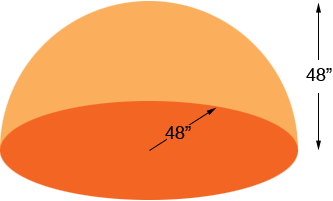SEARCH HOMEMath Central Quandaries & QueriesSubject: surface area of dome Name: chris Who are you: Other I need to figure the surface area of a dome which is 96" across and 48" tall. Could you help please? Thanks!Chris,

Since 48 is half of 96 it looks to me that your dome is half a sphere of radius 48"The surface area of a sphere is 4 π r2 where r is the radius of the sphere so the surface area of your dome is half of that.

Penny

Chris,

We know that another name for a dome is half of a sphere,
lets check, if the diameter was 96" accross then the radius has to be half that. Is it?
Let's try a similar dome,
38" accross, 19" high, taking the formula for surface area,

surface area = 4 π r2

we need to modify it because the dome will only be half of the sphere.
So,
Surface Area of a dome = (1/2) 4 π r2 = 2 π r2
We know radius (height) and pi, now we just need to sub the numbers in;
Surface Area of a dome = 2 π r2 = 2(3.1415...)(19)2
Hope this helps,
MelMath Central is supported by the University of Regina and The Pacific Institute for the Mathematical Sciences.